# Central Science: Chapter 8 Flashcards

Set Details Share
created 3 years ago by Chemistry4Life
3,438 views
College: First year, College: Second year, College: Third year, College: Fourth year
Subjects:
chemistry
Page to share:
Embed this setcancel
COPY
code changes based on your size selection
Size:
X

1

Which of the following has eight valence electrons?
A) Ti4+
B) Kr
C) Cl-
D) Na+
E) all of the above

E

2

Which of the following does not have eight valence electrons?
A) Ca+
B) Rb+
C) Xe
D) Br-
E) All of the above have eight valence electrons.

A

3

Lattice energy is ________.
A) the energy required to convert a mole of ionic solid into its constituent ions in the gas phase
B) the energy given off when gaseous ions combine to form one mole of an ionic solid
C) the energy required to produce one mole of an ionic compound from its constituent elements in their standard states
D) the sum of ionization energies of the components in an ionic solid
E) the sum of electron affinities of the components in an ionic solid

A

4

In ionic bond formation, the lattice energy of ions ________ as the magnitude of the ion charges ________ and the radii ________.
A) increases, decrease, increase
B) increases, increase, increase
C) decreases, increase, increase
D) increases, increase, decrease
E) increases, decrease, decrease

D

5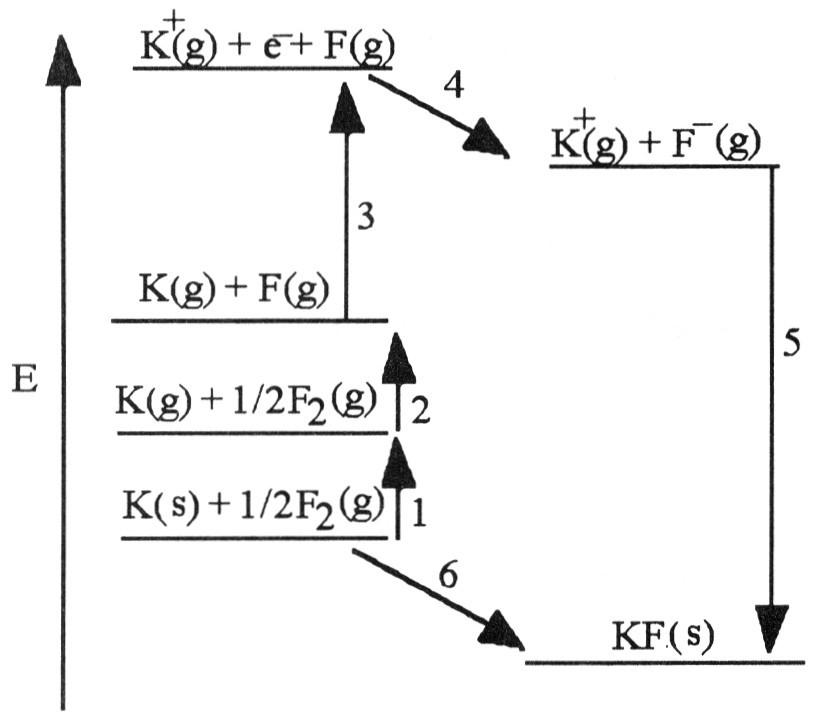The diagram below is the Born-Huber cycle for the formation of crystalline potassium fluoride.

5) Which energy change corresponds to the electron affinity of fluorine?
A) 2
B) 5
C) 4
D) 1
E) 6

C

6Which energy change corresponds to the first ionization energy of potassium?
A) 2
B) 5
C) 4
D) 3
E) 6

D

7

Using the Born-Haber cycle, the ΔH°f of KBr is equal to ________.
A) ΔH°f [K (g)] + ΔH°f [Br (g)] + I1(K) + E(Br) + ΔHlattice
B) ΔH°f [K (g)] - ΔH°f [Br (g)] - I1(K) - E(Br) - ΔHlattice
C) ΔH°f [K (g)] - ΔH°f [Br (g)] + I1(K) - E(Br) + ΔHlattice
D) ΔH°f [K (g)] + ΔH°f [Br (g)] - I1 - E(Br) + ΔHlattice
E) ΔH°f [K (g)] + ΔH°f [Br (g)] + I1(K) + E(Br) - ΔHlattice

E

8

The type of compound that is most likely to contain a covalent bond is ________.
A) one that is composed of a metal from the far left of the periodic table and a nonmetal from the far right of the periodic table
B) a solid metal
C) one that is composed of only nonmetals
D) held together by the electrostatic forces between oppositely charged ions
E) There is no general rule to predict covalency in bonds.

C

9

In which of the molecules below is the carbon-carbon distance the shortest?
A) H2C=CH2
B) H-C≡C-H
C) H3C-CH3
D) H2C=C=CH2
E) H3C-CH2-CH3

B

10

Of the molecules below, the bond in ________ is the most polar.
A) HBr
B) HI
C) HCl
D) HF
E) H2

D

11

Which of the following has the bonds correctly arranged in order of increasing polarity?
A) Be—F, Mg—F, N—F, O—F
B) O—F, N—F, Be—F, Mg—F
C) O—F, Be—F, Mg—F, N—F
D) N—F, Be—F, Mg—F, O—F
E) Mg—F, Be—F, N—F, O—F

B

12

Which of the following bonds would be considered non-polar covalent?
A) N-H
B) C-H
C) O-H
D) C-Cl
E) C-O

B

13

The Lewis structure of N2H2 shows ________.
A) a nitrogen-nitrogen triple bond
B) a nitrogen-nitrogen single bond
C) each nitrogen has one nonbonding electron pair
D) each nitrogen has two nonbonding electron pairs
E) each hydrogen has one nonbonding electron pair

C

14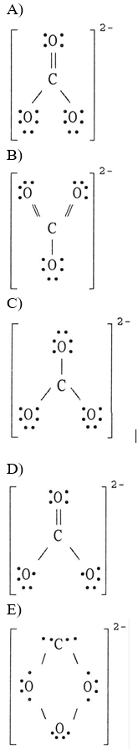The Lewis structure of the CO32- ion is ________.

A

15

How many electrons are in the Lewis structure of a nitrite ion (NO2-)?
A) 18
B) 17
C) 16
D) 23
E) 24

A

16

Resonance structures differ by ________.
A) number and placement of electrons
B) number of electrons only
C) placement of atoms only
D) number of atoms only
E) placement of electrons only

E

17

The oxidation number of iron in Fe2O3 is ________.
A) -2
B) +1
C) +3
D) +2
E) -3

C

18

To convert from one resonance structure to another, ________.
A) only atoms can be moved
B) electrons and atoms can both be moved
C) only electrons can be moved
D) neither electrons nor atoms can be moved

C

19

For ________ forms of a molecule or ion, the observed structure is an average of the ________ forms.
A) resonance, covalent
B) resonance, resonance
C) ionic, resonance
D) resonance, ionic
E) resonance, metallic

B

20

(i) NO2- (ii) NO3- (iii) SO3 2- (iv) SO4 2- (v) BrO3-

There can be two equivalent best resonance structures of ________.
A) (i)
B) (ii)
C) (iii)
D) (iv)
E) (v)

A

21

(i) NO2- (ii) NO3- (iii) SO3 2- (iv) SO4 2- (v) BrO3-

There can be three equivalent best resonance structures of ________.
A) (ii), (iii), and (v)
B) (i) and (ii)
C) (iii) and (v)
D) (iii), (iv), and (v)
E) all

A

22

A valid Lewis structure of ________ cannot be drawn without violating the octet rule.
A) NF3
B) IF3
C) PF3
D) SbF3
E) SO42-

B

23

Based on the octet rule, boron will most likely form a ________ ion.
A) B3-
B) B+
C) B3+
D) B2+
E) B2-

C

24

Which of the following does not have eight valence electrons?
A) Cl-
B) Xe
C) Ti+4
D) Rb+
E) Sr+

E

25

Which of the following Lewis structures would be an expansion to the octet rule?
A) SiF4
B) CF4
C) CCl4
D) PO43-
E) NF3

D

26

The central atom in ________ does not violate the octet rule.
A) SF4
B) KrF2
C) CF4
D) XeF4
E) ICl4-

C

27

Which of the following Lewis structures would be an expansion to the octet rule?
A) PH3
B) PCl3
C) CCl4
D) CO2
E) SO3

E

28

A valid Lewis structure of ________ cannot be drawn without violating the octet rule.
A) NI3
B) SO2
C) ICl5
D) SiF4
E) CO2

C

29

Which of the following Lewis structures would be an incomplete octet?
A) NF3
B) SO2
C) BCl3
D) CF4
E) SO32-

C

30

Why don't we draw double bonds between the Be atom and the Cl atoms in BeCl2?
A) That would give positive formal charges to the chlorine atoms and a negative formal charge to the beryllium atom.
B) There aren't enough electrons.
C) That would result in more than eight electrons around beryllium.
D) That would result in more than eight electrons around each chlorine atom.
E) That would result in the formal charges not adding up to zero.

A

31

Which of the following atoms may have an expansion to the octet?
A) P
B) C
C) H
D) O
E) B

A

32

Bond enthalpy is ________.
A) always positive
B) always negative
C) sometimes positive, sometimes negative
D) always zero
E) unpredictable

A

33

Given that the average bond energies for C-H and C-Br bonds are 413 and 276 kJ/mol, respectively, the heat of atomization of bromoform (CHBr3) is ________ kJ/mol.
A) 1241
B) 689
C) -689
D) 1378
E) -1378

A

34

Of the bonds C-C, C=C, and C≡C, the C-C bond is ________.
A) strongest/shortest
B) strongest/longest
C) weakest/longest
D) weakest/shortest
E) intermediate in both strength and length

C

35

) Of the bonds C-N, C=N, and C≡N, the C-N bond is ________.
A) strongest/shortest
B) strongest/longest
C) weakest/shortest
D) weakest/longest
E) intermediate in both strength and length

D

36

As the number of covalent bonds between two atoms increases, the distance between the atoms ________ and the strength of the bond between them ________.
A) increases, increases
B) decreases, decreases
C) increases, decreases
D) decreases, increases
E) is unpredictable, is unpredictable

D

37

Of the possible bonds between carbon atoms (single, double, and triple), ________.
A) a triple bond is longer than a single bond
B) a double bond is stronger than a triple bond
C) a single bond is stronger than a triple bond
D) a double bond is longer than a triple bond
E) a single bond is stronger than a double bond

D

38

Most explosives are compounds that decompose rapidly to produce ________ products and a great deal of ________.
A) gaseous, gases
B) liquid, heat
C) soluble, heat
D) solid, gas
E) gaseous, heat

E

39

Dynamite consists of nitroglycerine mixed with diatomaceous earth or cellulose. What is another name for dynamite?
A) KBr
B) KOH
C) TNT
D) C-4
E) CFC

C

40

________ is an explosive made of nitroglycerine and an absorbent such as diatomaceous earth.
A) KOH
B) TNT
C) C-4
D) KBr
E) CFC

B

41

Based on the octet rule, magnesium most likely forms a ________ ion.
A) Mg2+
B) Mg2-
C) Mg6-
D) Mg6+
E) Mg-

A

42

Based on the octet rule, phosphorus most likely forms a ________ ion.
A) P3+
B) P3-
C) P5+
D) P5-
E) P+

B

43

Based on the octet rule, aluminum most likely forms an ________ ion.
A) Al3+
B) Al4+
C) Al4-
D) Al+
E) Al-

A

44

Based on the octet rule, iodine most likely forms an ________ ion.
A) I2+
B) I4+
C) I4-
D) I+
E) I-

E

45

The electron configuration of the phosphide ion (P3-) is ________.
A) [Ne]3s2
B) [Ne]3s23p1
C) [Ne]3s23p3
D) [Ne]3p2
E) [Ne]3s23p6

E

46

The electron configuration of the sulfide ion (S2-) is ________.
A) [Ne]3s2
B) [Ne]3s23p1
C) [Ne]3s23p4
D) [Ne]3p2
E) [Ne]3s23p6

E

47

The halogens, alkali metals, and alkaline earth metals have ________ valence electrons, respectively.
A) 7, 4, and 6
B) 1, 5, and 7
C) 8, 2, and 3
D) 7, 1, and 2
E) 2, 7, and 4

D

48

The only noble gas without eight valence electrons is ________.
A) Ar
B) Ne
C) He
D) Kr
E) All noble gases have eight valence electrons.

C

49

Which of the following would have to lose two electrons in order to achieve a noble gas electron configuration?
O Sr Na Se Br

A) O, Se
B) Sr
C) Na
D) Br
E) Sr, O, Se

B

50

Which of the following would have to lose three electrons in order to achieve a noble gas electron configuration?
Si Mg Al Cl P

A) Si, P
B) Al
C) P
D) Cl
E) Mg, Al, P

B

51

Which of the following would have to gain two electrons in order to achieve a noble gas electron configuration?
O Sr Na Se Br

A) Br
B) Sr
C) Na
D) O, Se
E) Sr, O, Se

D

52

For a given arrangement of ions, the lattice energy increases as ionic radius ________ and as ionic charge ________.
A) decreases, increases
B) increases, decreases
C) increases, increases
D) decreases, decreases
E) This cannot be predicted.

A

53

For a given arrangement of ions, the lattice energy decreases as ionic radius ________ and as ionic charge ________.
A) decreases, increases
B) increases, decreases
C) increases, increases
D) decreases, decreases
E) This cannot be predicted.

B

54

The electron configuration of the S2- ion is ________.
A) [Ar]3s23p6
B) [Ar]3s23p2
C) [Ne]3s23p2
D) [Ne]3s23p6
E) [Kr]3s22p6

D

55

The electron configuration of the P3- ion is ________.
A) [Ar]3s23p6
B) [Ar]3s23p2
C) [Ne]3s23p6
D) [Ne]3s23p2
E) [Kr]3s22p6

C

56

What species has the electron configuration [Ar]3d2?
A) Mn2+
B) Cr2+
C) V3+
D) Fe3+
E) K+

C

57

What species has the electron configuration [Ar]3d4?
A) Mn2+
B) Cr2+
C) V3+
D) Fe3+
E) K+

B

58

What is the electron configuration for the Co2+ ion?
A) [Ar]4s13d6
B) [Ar]3d7
C) [Ar]3d5
D) [Ar]4s23d9
E) [Ne]3s23p10

B

59

What is the electron configuration for the Fe3+ ion?
A) [Ar]4s13d6
B) [Ar]4s03d7
C) [Ar]4s03d5
D) [Ar]4s23d9
E) [Ne]3s23p10

C

60

What is the electron configuration for the Cu2+ ion?
A) [Ar]4s13d10
B) [Ar]4s03d10
C) [Ar]4s03d9
D) [Ar]4s23d9
E) [Ar]4s03d11

C

61

The formula of palladium (IV) sulfide is ________.
A) Pd2S4
B) PdS4
C) Pd4S
D) PdS2
E) Pd2S2

D

62

Elements from opposite sides of the periodic table tend to form ________.
A) covalent compounds
B) ionic compounds
C) compounds that are gaseous at room temperature
D) homonuclear diatomic compounds
E) covalent compounds that are gaseous at room temperature

B

63

Determining lattice energy from Born-Haber cycle data requires the use of ________.
A) the octet rule
B) Coulomb's law
C) Periodic law
D) Hess's law

D

64

A ________ covalent bond between the same two atoms is the longest.
A) single
B) double
C) triple
D) strong
E) They are all the same length.

A

65

How many hydrogen atoms must bond to silicon to give it an octet of valence electrons?
A) 1
B) 2
C) 3
D) 4
E) 5

D

66

A double bond consists of ________ pairs of electrons shared between two atoms.
A) 1
B) 2
C) 3
D) 4
E) 6

B

67

A triple bond consists of ________ pairs of electrons shared between two atoms.
A) 1
B) 2
C) 3
D) 4
E) 6

C

68

What is the maximum number of double bonds that a hydrogen atom can form?
A) 0
B) 1
C) 2
D) 3
E) 4

A

69

What is the maximum number of double bonds that a carbon atom can form?
A) 4
B) 1
C) 0
D) 2
E) 3

D

70

What is the maximum number of triple bonds that a carbon atom can form?
A) 4
B) 1
C) 0
D) 2
E) 3

B

71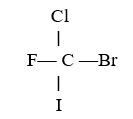In the molecule below, which atom has the largest partial negative charge?

A) Cl
B) F
C) Br
D) I
E) C

B

72

The ability of an atom in a molecule to attract electrons is best quantified by the ________.
A) paramagnetism
B) diamagnetism
C) electronegativity
D) electron charge-to-mass ratio
E) first ionization potential

C

73

Given the electronegativities below, which covalent single bond is most polar?
Element: H C N O
Electronegativity: 2.1 2.5 3.0 3.5

A) C—H
B) N—H
C) O—H
D) O—C
E) O—N

C

74

Electronegativity ________ from left to right within a period and ________ from top to bottom within a group.
A) decreases, increases
B) increases, increases
C) increases, decreases
D) stays the same, increases
E) increases, stays the same

C

75

Electropositivity ________ from left to right within a period and ________ from top to bottom within a group.
A) decreases, increases
B) increases, increases
C) increases, decreases
D) stays the same, increases
E) increases, stays the same

A

76

A nonpolar bond will form between two ________ atoms of ________ electronegativity.
A) different, opposite
B) identical, different
C) different, different
D) similar, different
E) identical, equal

E

77

The ion ICl4- has ________ valence electrons.
A) 34
B) 35
C) 36
D) 28
E) 8

C

78

The ion NO- has ________ valence electrons.
A) 15
B) 14
C) 16
D) 10
E) 12

E

79

The ion PO43- has ________ valence electrons.
A) 14
B) 24
C) 27
D) 29
E) 32

E

80

The Lewis structure of AsH3 shows ________ nonbonding electron pair(s) on As.
A) 0
B) 1
C) 2
D) 3
E) This cannot be determined from the data given.

B

81

The Lewis structure of PF3 shows that the central phosphorus atom has ________ nonbonding and ________ bonding electron pair(s).
A) 2, 2
B) 1, 3
C) 3, 1
D) 1, 2
E) 3, 3

B

82

The Lewis structure of HCN (H bonded to C) shows that ________ has ________ nonbonding electron pair(s).
A) C, 1
B) N, 1
C) H, 1
D) N, 2
E) C, 2

B

83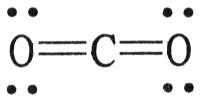The formal charge on carbon in the molecule below is ________.

A) 0
B) +1
C) +2
D) +3
E) -1

A

84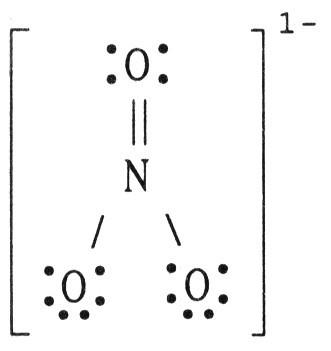The formal charge on nitrogen in NO3- is ________, where the Lewis structure of the ion is:

A) -1
B) 0
C) +1
D) +2
E) -2

C

85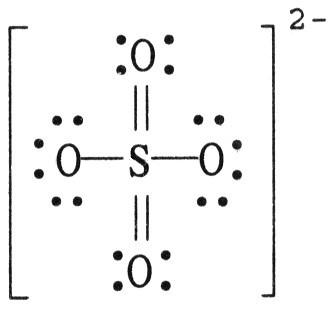The formal charge on sulfur in SO42- is ________, where the Lewis structure of the ion is:

A) -2
B) 0
C) +2
D) +4
E) -4

B

86

In the Lewis structure of ClF, the formal charge on Cl is ________, and the formal charge on F is ________.
A) -1, -1
B) 0, 0
C) 0, -1
D) +1, -1
E) -1, +1

B

87

In the Lewis structure of HCO3-, the formal charge on H is ________, and the formal charge on C is ________.
A) -1, -1
B) 0, 0
C) 0, -1
D) +1, -1
E) -1, +1

B

88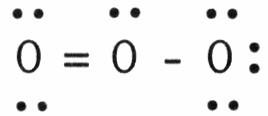In the resonance form of ozone shown below, the formal charge on the central oxygen atom is ________.

A) 0
B) +1
C) -1
D) +2
E) -2

B

89

How many equivalent resonance forms can be drawn for CO32-? (Carbon is the central atom.)
A) 1
B) 2
C) 3
D) 4
E) 0

C

90

How many equivalent resonance forms can be drawn for the nitrate ion?
A) 0
B) 2
C) 3
D) 4
E) 1

C

91

How many equivalent resonance structures can be drawn for the molecule of SO3 without having to violate the octet rule on the sulfur atom?
A) 5
B) 2
C) 1
D) 4
E) 3

E

92

How many different types of resonance structures can be drawn for the ion SO32-?
A) 1
B) 2
C) 3
D) 4
E) 5

C

93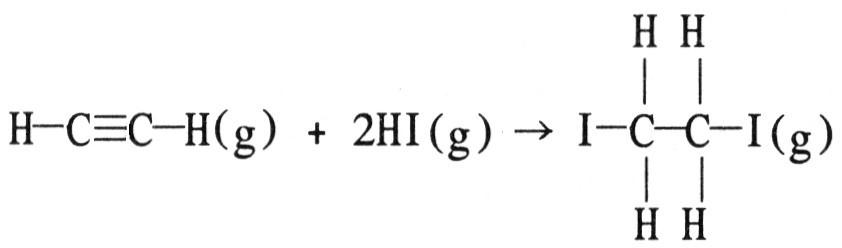Using the table of average bond energies below, the ΔH for the reaction is ________ kJ.

Bond: C≡C C-C H-I C-I C-H
D (kJ/mol): 839 348 299 240 413

A) +160
B) -160
C) -217
D) -63
E) +63

C

94

Using the table of average bond energies below, the ΔH for the reaction is ________ kJ.

H-C≡C-H (g) + H-Cl (g) → H2C=CHCl (g)
Bond: C≡C C=C H-Cl C-Cl C-H
D (kJ/mol): 839 614 431 328 413

A) +741
B) -85
C) -839
D) -741
E) +85

B

95

Using the table of average bond energies below, the △H for the reaction is ________ kJ.

H3C-O-H (g) → C≡O (g) + 2H2 (g)
Bond: C-O C=O C≡O C-H H-H O-H
D (kJ/mol): 358 799 1072 413 436 463

A) +276
B) -276
C) +116
D) +735
E) -116

C

96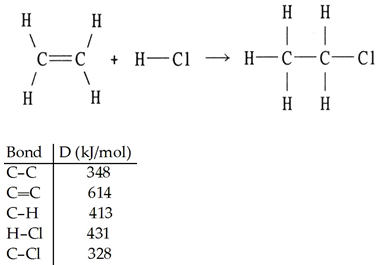Using the table of bond dissociation energies, the ΔH for the following gas-phase reaction is ________ kJ.

A) -44
B) 38
C) 304
D) 2134
E) -38

A

97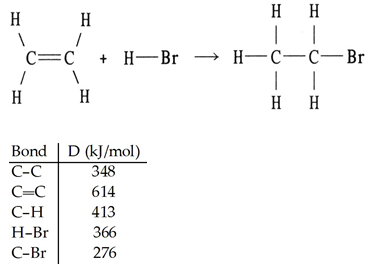Using the table of bond dissociation energies, the ΔH for the reverse of following gas-phase reaction is ________ kJ.

A) +57
B) +291
C) 2017
D) -57
E) -291

A

98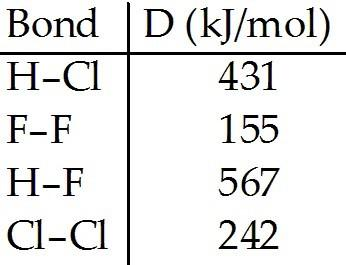Using the table of bond dissociation energies, the ΔH for the following reaction is ________ kJ.
2HCl (g) + F2 (g) → 2HF (g) + Cl2 (g)

A) -359
B) -223
C) 359
D) 223
E) 208

A

99

There are ________ paired and ________ unpaired electrons in the Lewis symbol for a fluorine atom.
A) 4, 2
B) 4, 1
C) 6, 1
D) 0, 5
E) 2, 5

C

100

The ________ ion has a noble gas electron configuration.
A) Be2+
B) Li2+
C) Li
D) Mg2-
E) Al2+

A

101

The ________ ion has a noble gas electron configuration.
A) Cl-
B) F2-
C) F
D) S2+
E) O-

A

102

The ________ ion has eight valence electrons.
A) Sc3+
B) Ti3+
C) V3+
D) Cr3+
E) Mn3+

A

103

There are ________ unpaired electrons in the Lewis symbol for an oxygen atom .
A) 0
B) 1
C) 2
D) 4
E) 3

C

104

What is the principal quantum number of the last shell for the element barium?
A) 5
B) 4
C) 3
D) 6
E) 2

D

105

The oxide of which of the following metals should have the greatest lattice energy?
A) calcium
B) strontium
C) magnesium
D) beryllium
E) barium

D

106

The ________ ion is represented by the electron configuration [Ar]3d2.
A) Cr4+
B) V4+
C) Ti4+
D) Mn4+
E) Sc4+

A

107

Which of the following species does the noble gas electron configuration [Kr]4d10 represent?
A) Cd
B) Cd+
C) Ag+
D) Ag2+
E) Sr2+

C

108

Which of the following noble gas electron configurations represents the Ru+ cation?
A) [Kr]5s24d5
B) [Kr]5s24d7
C) [Kr]4d5
D) [Ar]4s13d6
E) [Kr]5s14d6

E

109

Ni2+ ions are represented by the electron configuration ________.
A) [Ar]3d8
B) [Ar]3d10
C) [Ar]3d6
D) [Ar]4s23d6
E) [Kr]4d8

A

110

How many single covalent bonds must a chlorine atom form to have a complete octet in its valence shell?
A) 0
B) 1
C) 2
D) 3
E) 4

B

111

The most electronegative atom of the ones listed below is ________.
A) B
B) Al
C) Ga
D) In
E) Tl

A

112

Of the atoms below, ________ is the most electronegative.
A) Ba
B) Sr
C) Ca
D) Mg
E) Be

E

113

Of the atoms below, ________ is the most electronegative.
A) C
B) Si
C) Ge
D) B
E) Al

A

114

Of the atoms below, ________ is the least electronegative.
A) B
B) F
C) C
D) N
E) O

A

115

Of the bonds below, ________ is the least polar.
A) C-O
B) N-O
C) C-F
D) S-O
E) K-Br

B

116

Which two bonds are most similar in polarity?
A) O-F and Cl-F
B) B-F and Cl-F
C) Al-Cl and I-Br
D) I-Br and Si-Cl
E) C-Cl and Be-Cl

A

117

There are ________ valence electrons in the Lewis structure of CH3Cl.
A) 14
B) 16
C) 18
D) 20
E) 22

A

118

How many valence electrons are in the Lewis structure of CH3OCH3.?
A) 16
B) 18
C) 20
D) 24
E) 22

C

119

In the Lewis symbol for a nitrogen atom, there are ________ paired and ________ unpaired electrons.
A) two, three
B) one, three
C) three, two
D) zero, five
E) two, two

A

120

The oxidation number of phosphorus in PF5 is ________.
A) +5
B) +3
C) +1
D) -5
E) 0

A

121

The central atom in ________ violates the octet rule.
A) PF5
B) SF2
C) Cl2
D) Br2CO
E) FCN

A

122

Of the following, ________ cannot accommodate more than an octet of electrons.
A) Ni
B) Sc
C) Be
D) Os
E) Sb

C

123

How many covalent bonds are in the Lewis Structure of CH3CHCl2.?
A) 6
B) 8
C) 7
D) 5
E) 4

C

124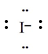The electron configuration that corresponds to the Lewis symbol, [see img] is ________.

[Ne]3s23p6

125

Write the balanced chemical equation for the reaction for which △H°rxn is the lattice energy for sodium chloride.

NaCl (s) → Na+ (g) + Cl- (g)

126

Using the noble gas shorthand notation, write the electron configuration for Fe2+.

[Ar]3d6

127

Give the electron configuration of Zn2+.

[Ar]3d10

128

Which halogen, bromine or iodine, will form the more polar bond with phosphorus?

bromine

129

Draw the Lewis structure of ICl2+.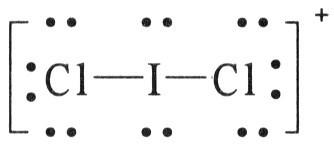130

) If more than one Lewis structure can be drawn then the molecule or ion is said to have ________ forms.

resonance

131

How many resonance forms exist for benzene?

two

132

If the bonds in the reactants of a reaction are weaker than the bonds in the product, the reaction is ________.

exothermic

133

Which two elements in period 2 form compounds that can violate the octet rule?

boron and beryllium

134

Polyatomic ions with an even number of electrons will follow the ________ rule.

octet

135

The strength of a ________ bond is measured by its bond enthalpy.

covalent

136

An exothermic reaction should have ________ chemical bonds and decompose to a molecule with ________ bonds.

weak, strong

137

Calculate the bond energy of C—F given that the heat of atomization of CHFClBr is 1502 kJ/mol, and that the bond energies of C—H, C—Br, and C—Cl are 413, 276, and 328 kJ/mol, respectively.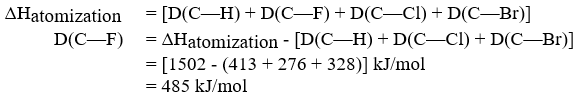138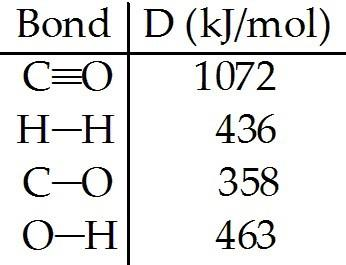The reaction below is used to produce methanol:

CO (g) + 2H2 (g) → CH3OH (l) △Hrxn = -128 kJ

(a) Calculate the C—H bond energy given the following data: [see img]

(b) The tabulated value of the (C-H) bond energy is 413 kJ/mol. Explain why there is a difference between the number you have calculated in (a) and the tabulated value.

(a)
△Hrxn = D(C≡O) + 2 D(H-H) - [3 D(C-H) + D(C-O) + D(O-H)]
3 D(C-H) = -△Hrxn + D(C≡O) + 2 D(H-H) - D(C-O) - D(O-H)
D(C-H) = (128 + 1072 + 2(436) - 358 - 463)/3 = 417
D(C-H) = 417 kJ/mol

(b)
Tabulated values, like those in Table 8.4, are averaged from many bond energies measured for C—H bonds in many different molecules.

139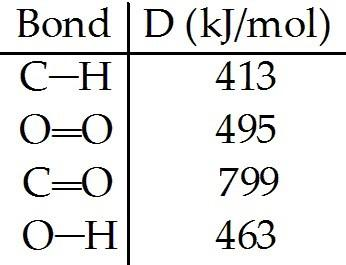From the information given below, calculate the heat of combustion of methane. Start by writing the balanced equation.

CH4 + 2O2 → CO2 + 2H2O
ΔHcombustion = (4 mol C—H)(DC—H) + (2 mol O O)(DO O)
- [(2 mol C O)(DC O) - (4 mol O—H)(DO—H)]
= [(4 × 413 + 2 × 495) - (2 × 799 + 4 × 463)] kJ
ΔHcombustion = -808 kJ

140

Atoms surrounded by eight valence electrons tend to lose electrons.

false

141

The greater the lattice energy, the greater the charges on the participatory ions and the smaller their radii.

true

142

Most transition metals do not form ions with a noble gas configuration.

true

143

When a metal gains an electron, the process is endothermic.

false

144

Electron affinity is a measure of how strongly an atom can attract additional electrons.

true

145

As electronegativity difference increases, bond length will decrease.

true

146

In some molecules and polyatomic ions, the sum of the valence electrons is odd and as a result the octet rule fails.

true

147

A positive change in bond enthalpy is required to break a bond.

true